# Proportions Ratios and Proportions Ratios and Proportions Ratios

• Slides: 15Proportions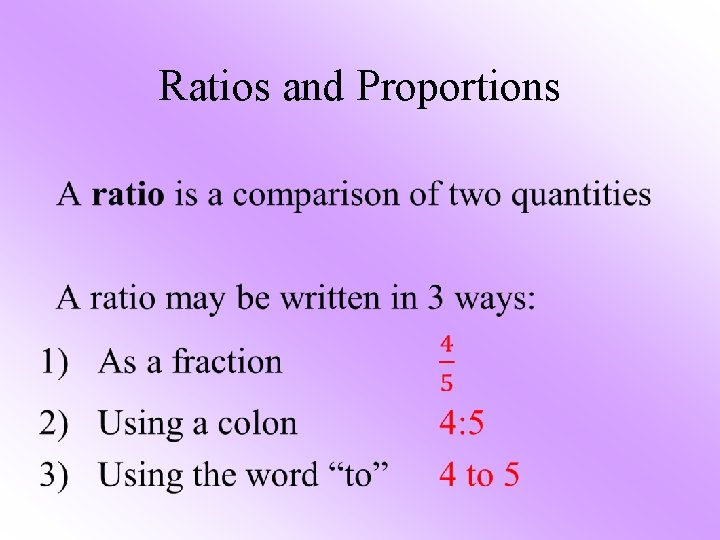Ratios and Proportions •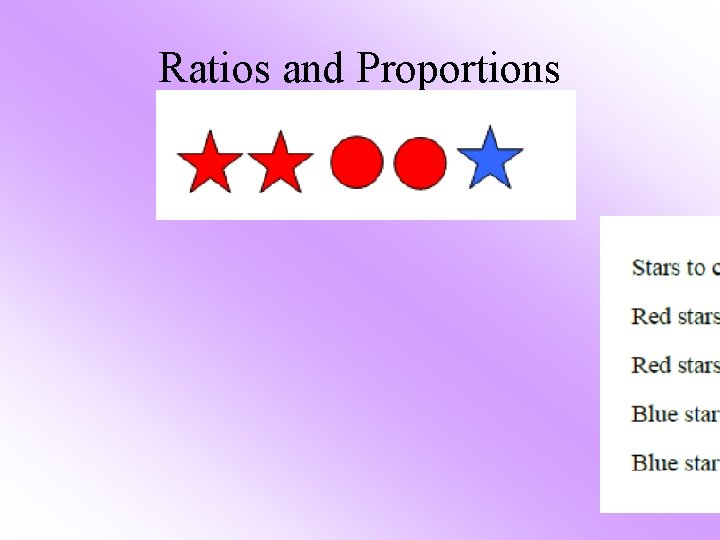Ratios and Proportions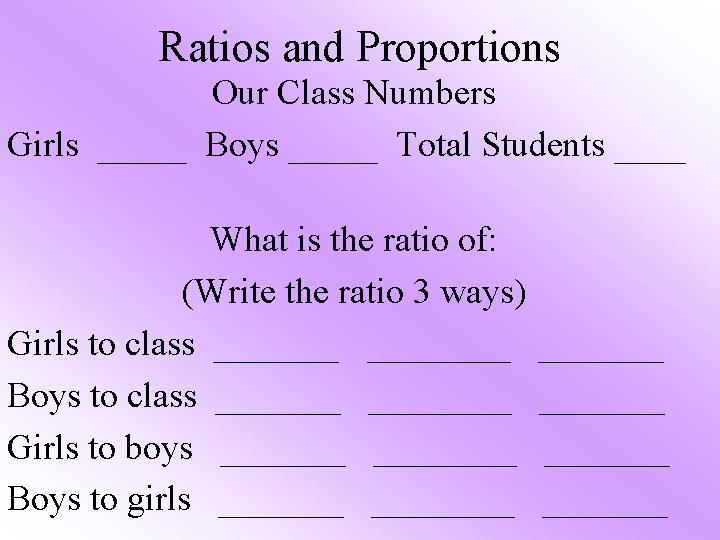Ratios and Proportions Our Class Numbers Girls _____ Boys _____ Total Students ____ What is the ratio of: (Write the ratio 3 ways) Girls to class ________ Boys to class ________ Girls to boys ________ Boys to girls ________ _______Ratios and Proportions 1. What is the ratio in simplest form of the number of circles to the number of triangles? Express the ratio 3 different ways. ● ●▲ ▲ ● ▲▲ ● ▲ ▲Ratios and Proportions 2. What is the ratio, in simplest form, of the number of squares to the total number of quadrilaterals? Express the ratio 3 different ways █ █ ■■ █ ■ █ █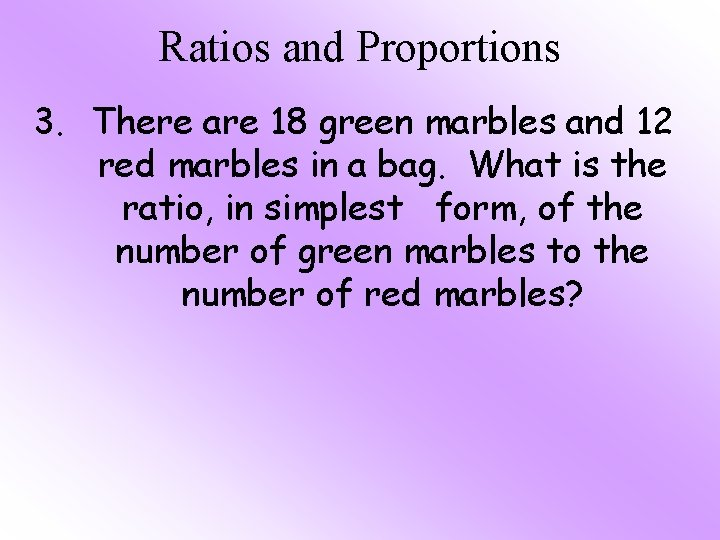Ratios and Proportions 3. There are 18 green marbles and 12 red marbles in a bag. What is the ratio, in simplest form, of the number of green marbles to the number of red marbles?Ratios and Proportions 4. There are 36 girls and 24 boys in the school chorus. In three different ways, write the ratio, in simplest form, of the number of girls to the number of students in the chorus.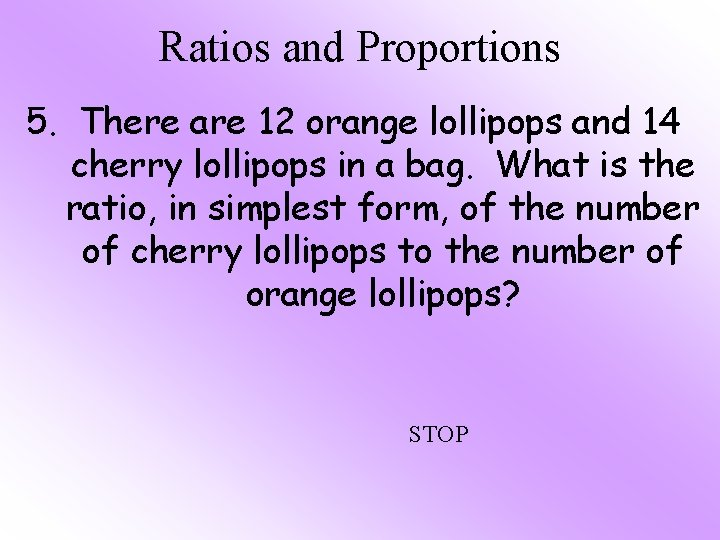Ratios and Proportions 5. There are 12 orange lollipops and 14 cherry lollipops in a bag. What is the ratio, in simplest form, of the number of cherry lollipops to the number of orange lollipops? STOP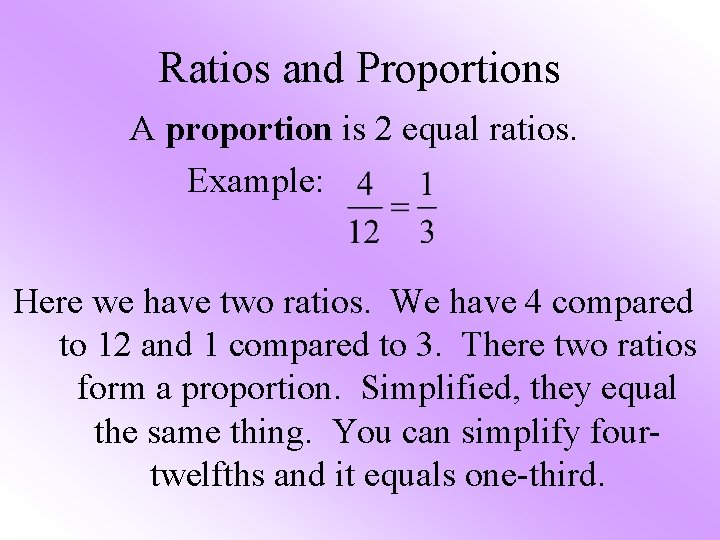Ratios and Proportions A proportion is 2 equal ratios. Example: Here we have two ratios. We have 4 compared to 12 and 1 compared to 3. There two ratios form a proportion. Simplified, they equal the same thing. You can simplify fourtwelfths and it equals one-third.Ratios and Proportions To determine if two ratios form a proportion: 1. Reduce or multiply one (or both) of the ratios in order to get common denominators 2. Once you have common denominators, see if the numerators are the same. If both ratios match perfectly, they are proportions!Determine Whether each pair of ratios forms a proportionDetermine Whether each pair of ratios forms a proportionDetermine Whether each pair of ratios forms a proportion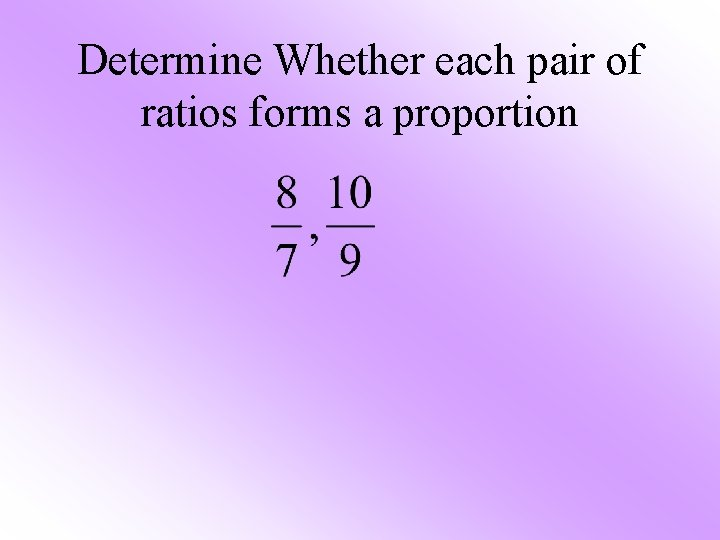Determine Whether each pair of ratios forms a proportion